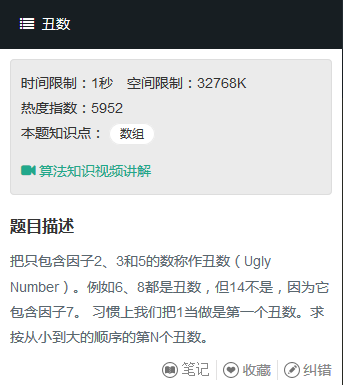# 牛客：剑指offer：丑数（Java）public class Solution {
public int GetUglyNumber_Solution(int index) {
if(index <= 0)
return 0;
int[] ugly = new int[index];
ugly = 1;
int i2 = 0;
int i3 = 0;
int i5 = 0;
for(int i = 1; i < index; i++){
int tmp = min(ugly[i2]*2,ugly[i3]*3,ugly[i5]*5);
ugly[i] = tmp;
while(ugly[i2]*2 <= ugly[i])
i2++;
while(ugly[i3]*3 <= ugly[i])
i3++;
while(ugly[i5]*5 <= ugly[i])
i5++;
}
return ugly[index-1];
}
public int min(int a,int b,int c){
if(a > b)
a = b;
if(a > c)
a = c;
return a;
}
}